# RD Sharma Solutions Class 8 Chapter 26 Data Handling IV (Probability) Exercise 26.1

Here you can get free RD Sharma Solutions for Class 8 Maths Chapter 26 Data Handling IV (Probability) Exercise 26.1. All RD Sharma Book Solutions are given here exercise wise for the chapter Data Handling IV (Probability). RD Sharma Solutions are helpful in the preparation of several school level, graduate and undergraduate level competitive exams. Practicing questions from RD Sharma Mathematics Solutions for Class 8 Chapter 26 Data Handling IV (Probability) is proven to enhance your math skills.

 Class: Class 8th Chapter: Chapter 26 Exercise: Exercise 26.1 Name: Data Handling IV (Probability)

## RD Sharma Solutions Class 8 Chapter 26 Data Handling IV (Probability) Exercise 26.1

RD Sharma Class 8 Solutions Chapter 26 for Data Handling IV (Probability) Exercise 26.1 are given below.

RD Sharma Solutions Class 8 Chapter 26 Data Handling IV Probability Exercise 26.1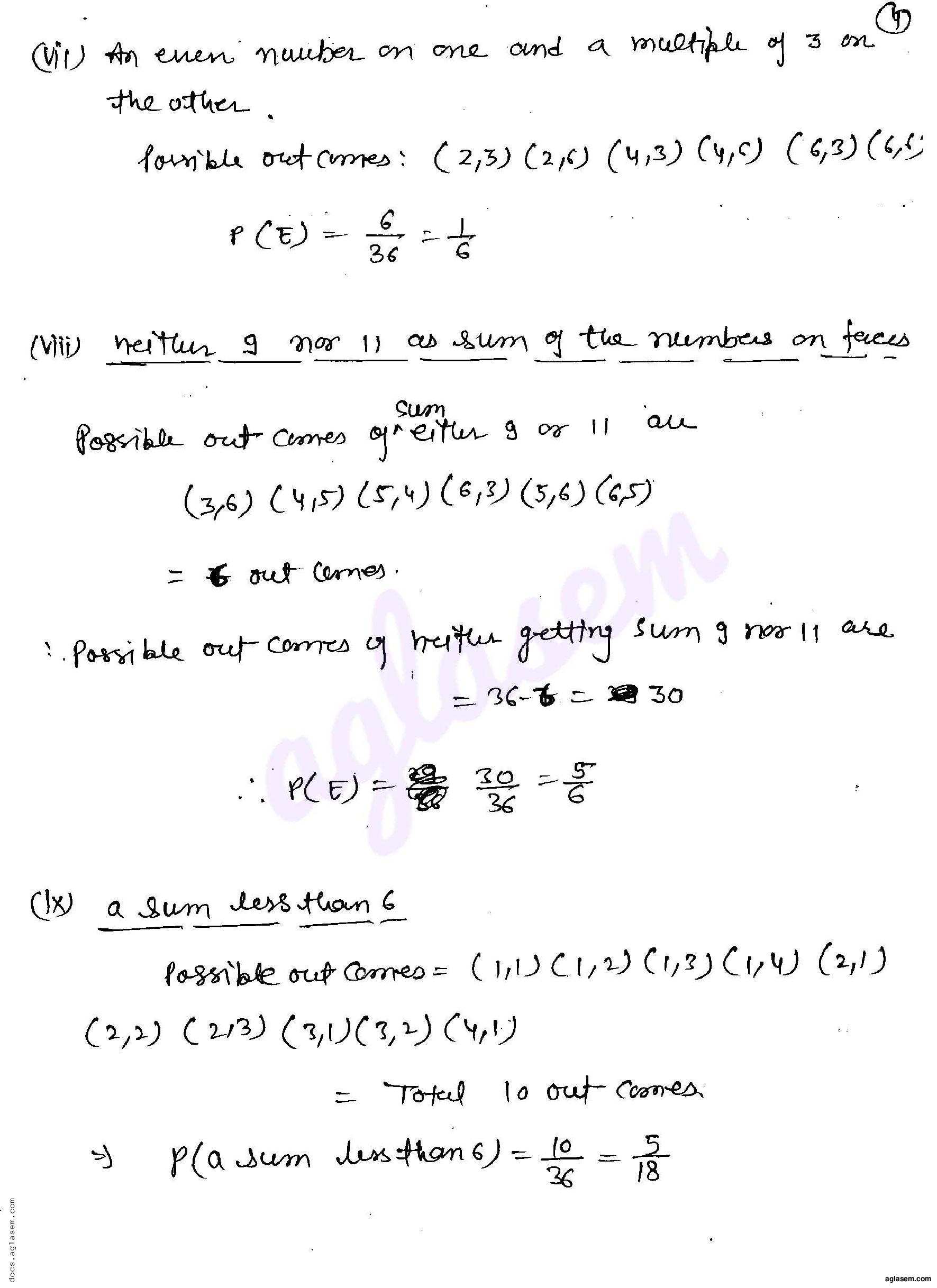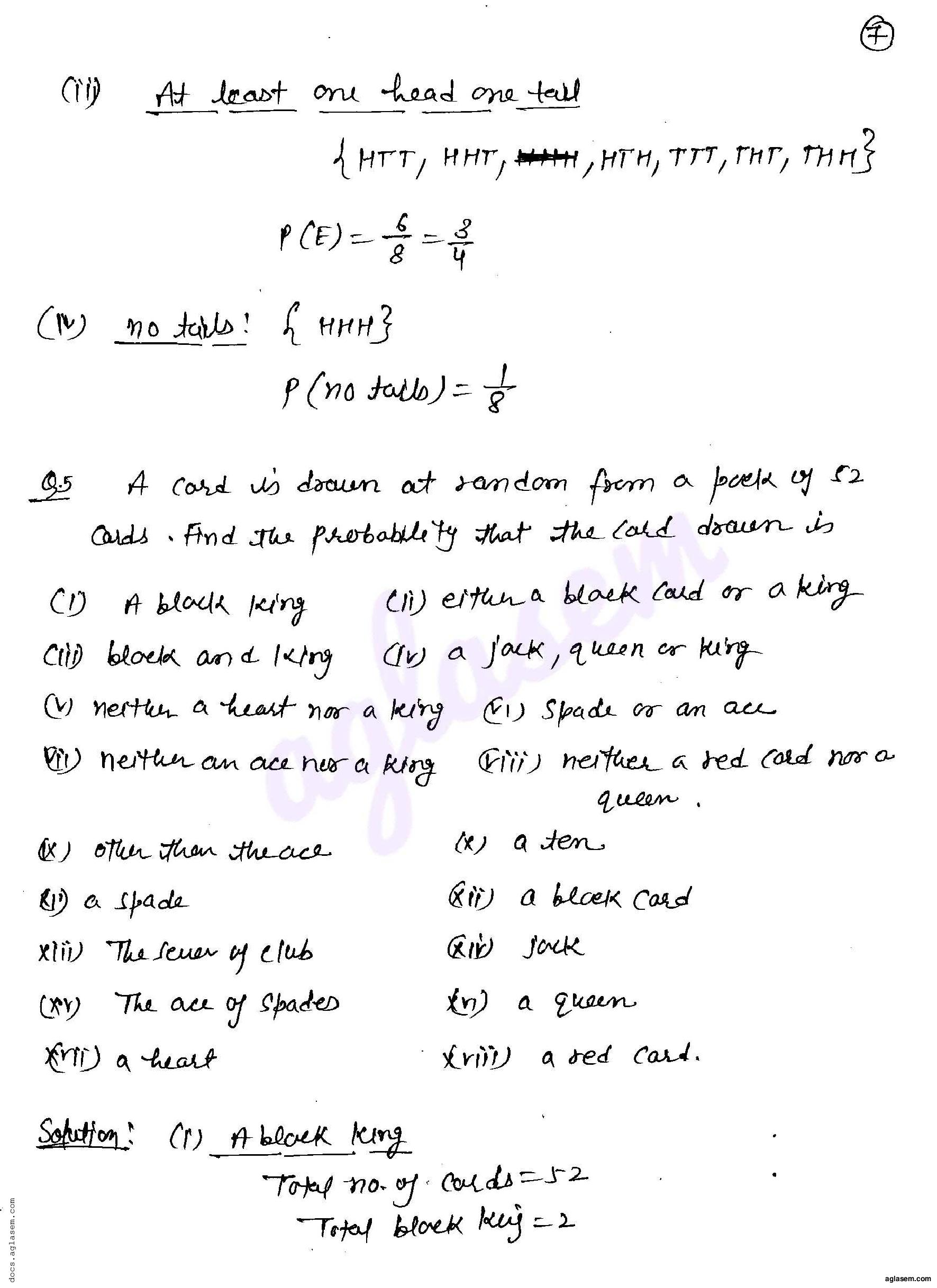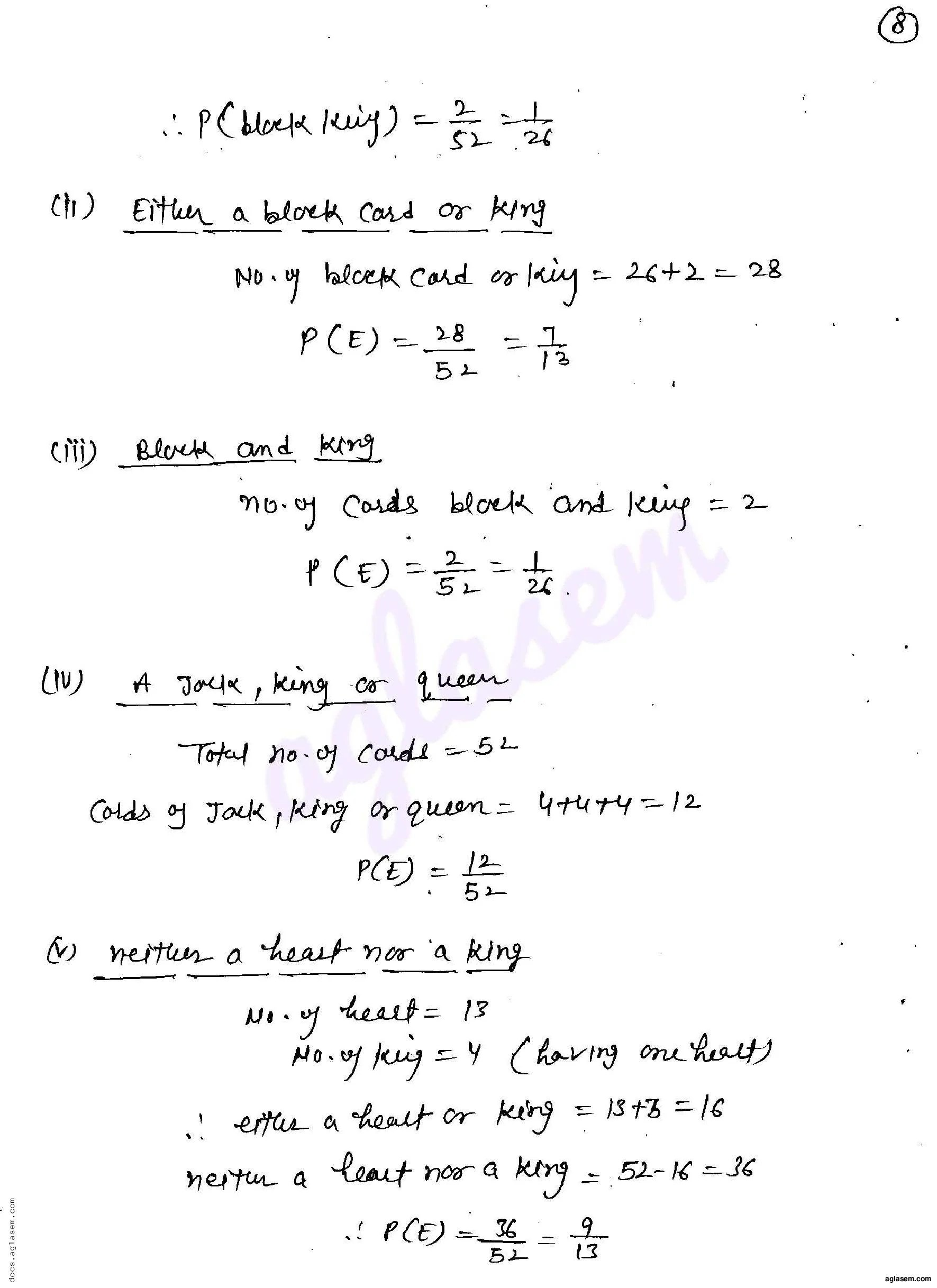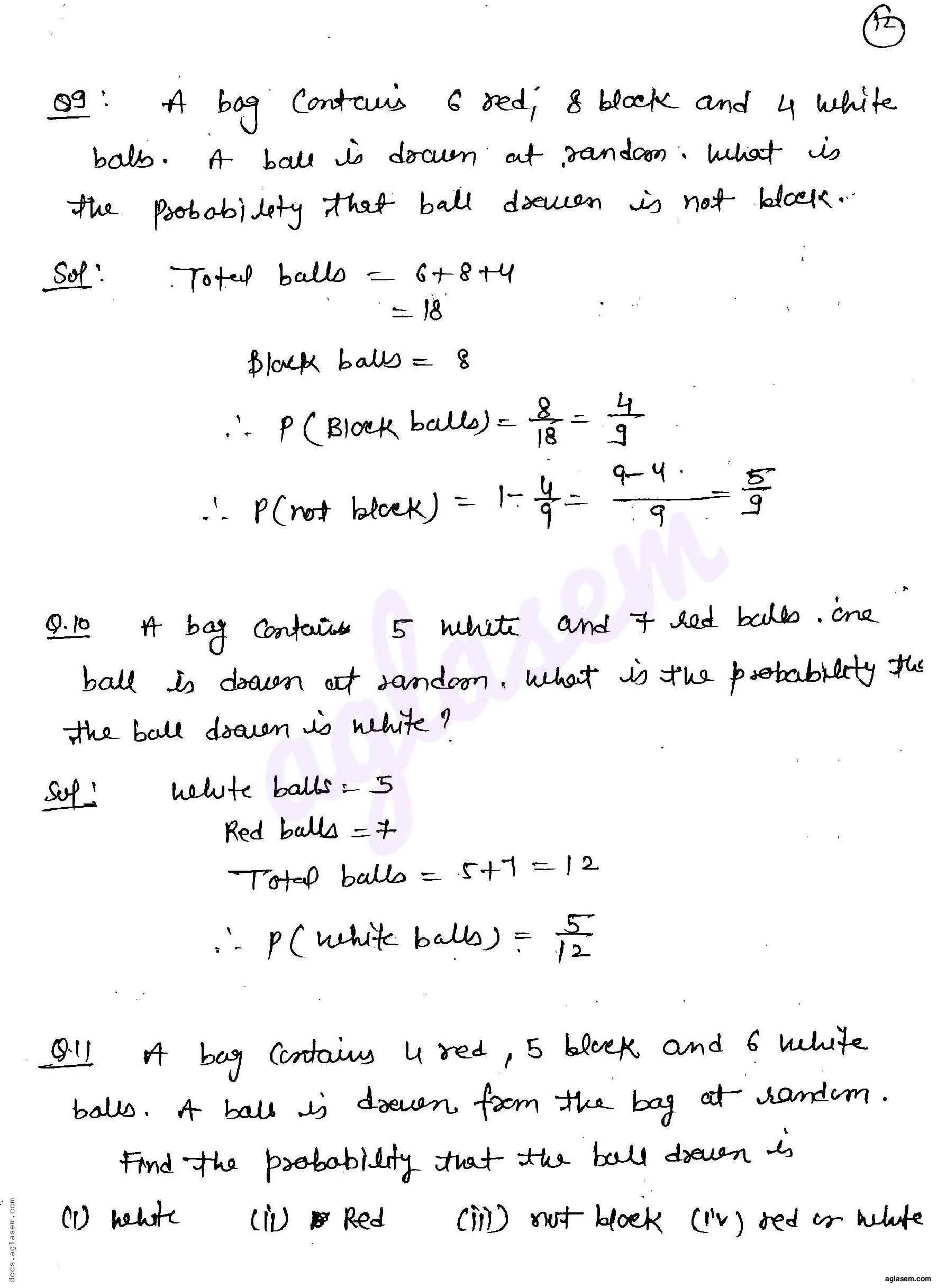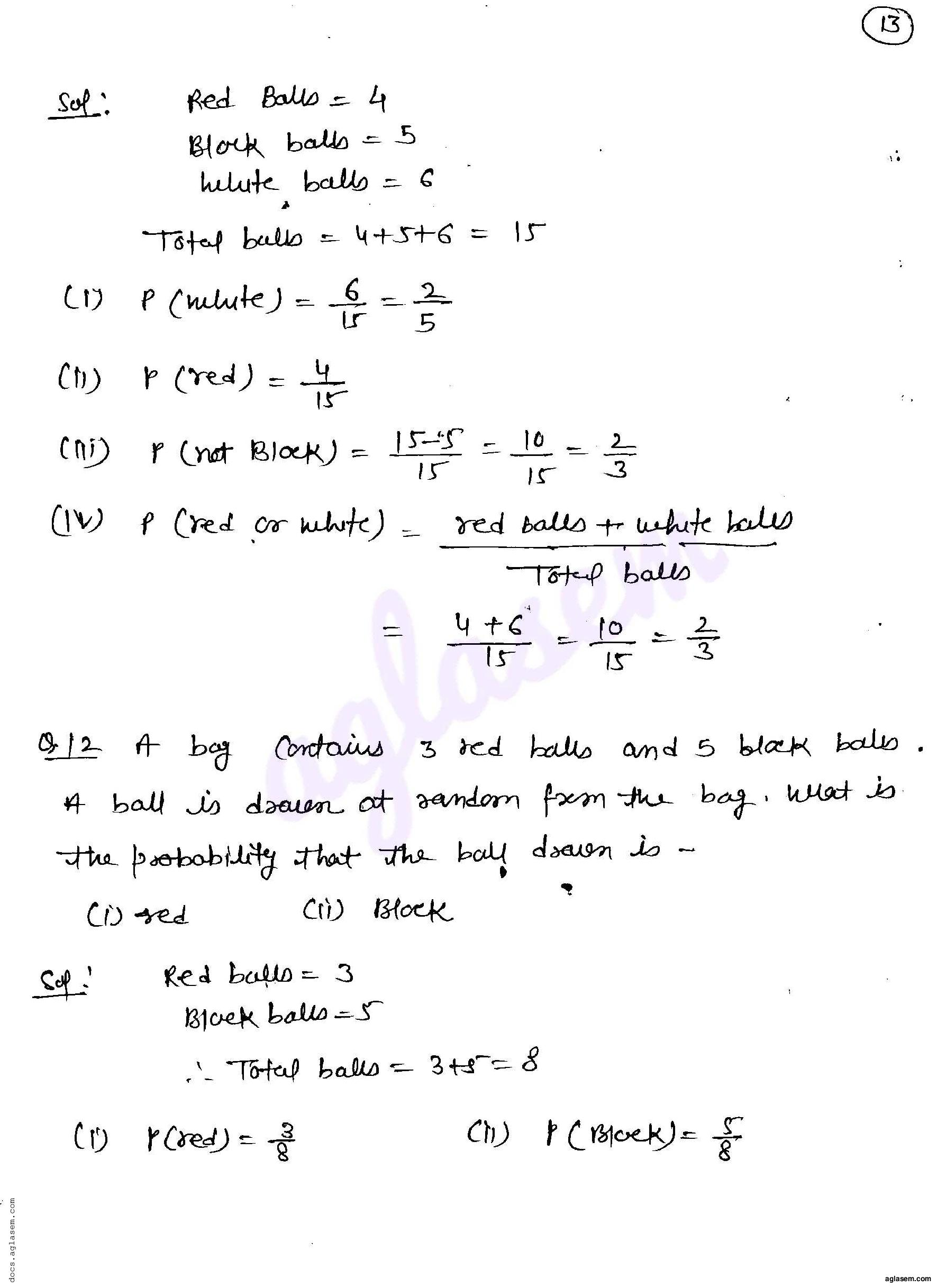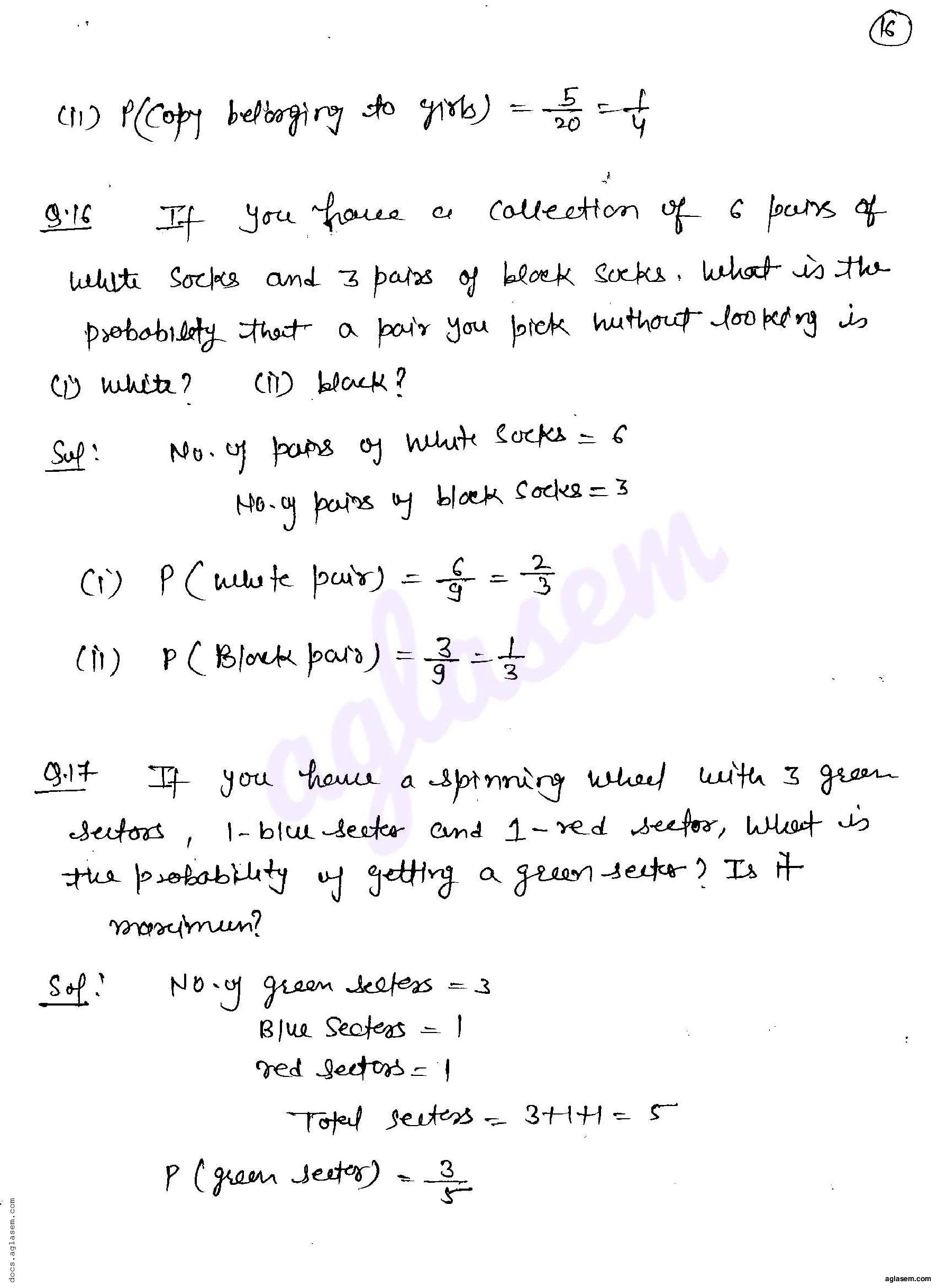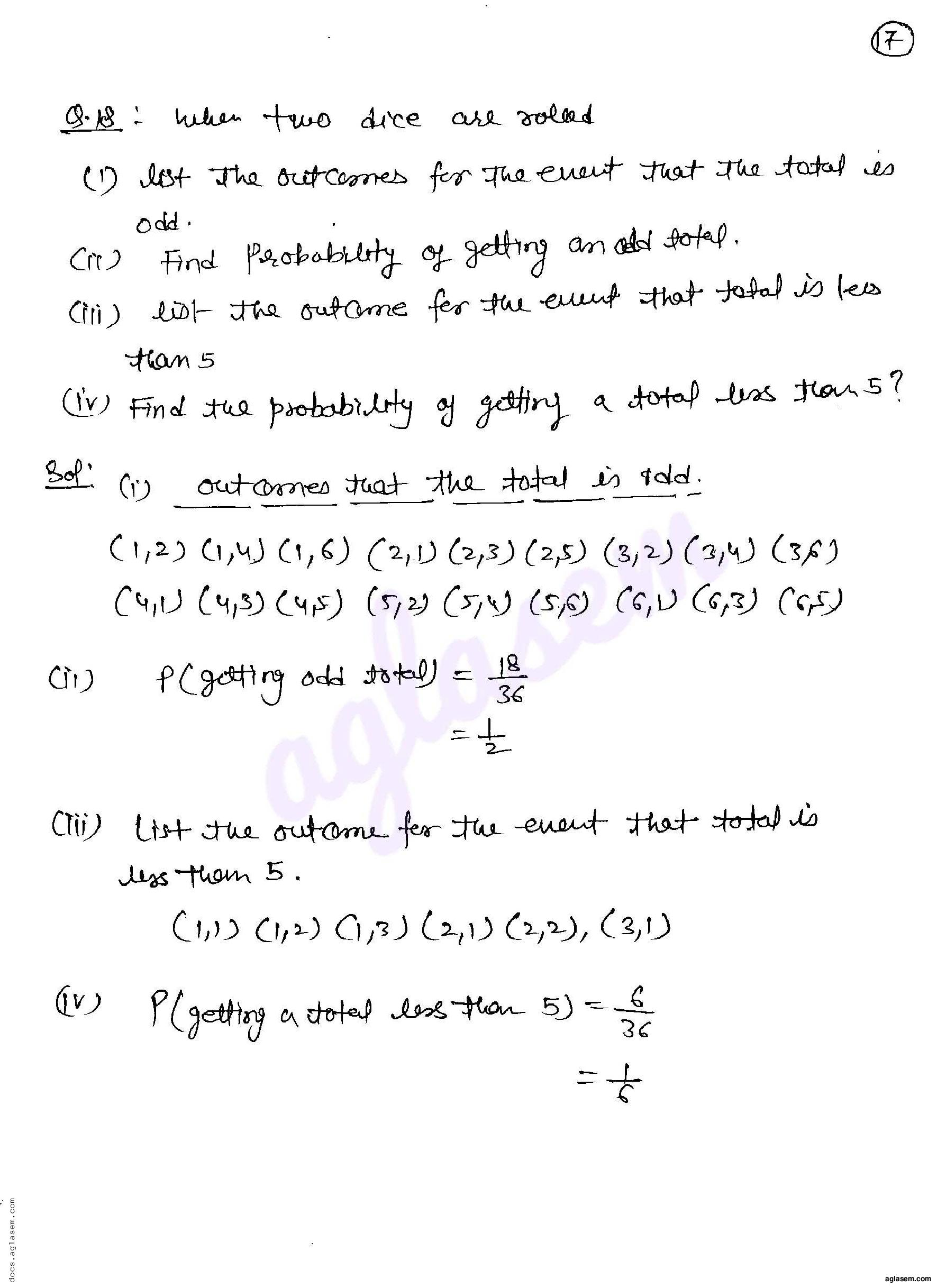RD Sharma Solutions for Class 8 Maths PDF download for Chapter 26 Data Handling IV (Probability) Exercise 26.1 is available here for free.

 « Previous Next »

### RD Sharma Solutions Class 8 Chapter 26 Exercise Wise

You can also see RD Sharma Solutions of all exercises of Chapter 26 – Data Handling IV (Probability) from here.

### RD Sharma Solutions Class 8 Chapter Wise

If you want RD Sharma solutions of any topic other than Chapter 26 Data Handling IV (Probability), check it from here. Mathematics by RD Sharma has all chapters that are in your Class 8 syllabus.

Click to rate this post!
[Total: 2 Average: 5]